## Audio

[Audio][slideshow]

## A complete basic tutorial of 555 Timer IC.

This article covers every basic aspect of 555 Timer IC. You may already know that SE/NE 555 is a Timer IC introduced by Signetics corporation in 1970’s. In this article we cover the following information about 555 Timer IC.

### 5. Working Principle

If you still need a detailed understanding of the 555 timer IC, we have reviewed 3 books in our online store. These books covers all the aspects of the 555 timer IC, along with its applications. To get reviews and to buy them, click here:- 3 Great Books to Learn 555 Timer Circuits and Projects

## 1. Introduction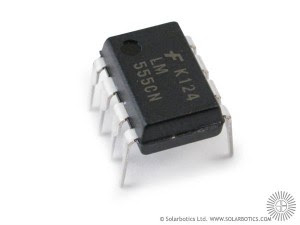555 timer IC

The 555 timer IC was introduced in the year 1970 by Signetic Corporation and gave the name SE/NE 555 timer. It is basically a monolithic timing circuit that produces accurate and highly stable time delays or oscillation. When compared to the applications of an op-amp in the same areas, the 555IC is also equally reliable and is cheap in cost. Apart from its applications as a monostable multivibrator and astable multivibrator, a 555 timer can also be used in dc-dc converters, digital logic probes, waveform generators, analog frequency meters and tachometers, temperature measurement and control devices, voltage regulators etc. The timer IC is setup to work in either of the two modes – one-shot or monostabl or as a free-running or astable multivibrator.The SE 555 can be used for temperature ranges between – 55°C to 125° . The NE 555 can be used for a temperature range between 0° to 70°C.

### The important features of the 555 timer are :

• It operates from a wide range of power supplies ranging from + 5 Volts to + 18 Volts supply voltage.
• Sinking or sourcing 200 mA of load current.
• The external components should be selected properly so that the timing intervals can be made into several minutes along with the frequencies exceeding several hundred kilo hertz.
• The output of a 555 timer can drive a transistor-transistor logic (TTL) due to its high current output.
• It has a temperature stability of 50 parts per million (ppm) per degree Celsius change in temperature, or equivalently 0.005 %/°C.
• The duty cycle of the timer is adjustable.
• The maximum power dissipation per package is 600 mW and its trigger and reset inputs has logic compatibility. More features are listed in the datasheet.

## 2. IC Pin Configuration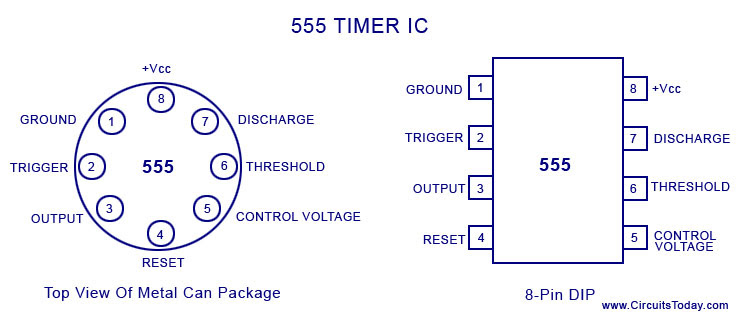555 Timer IC Pin Configuration

The 555 Timer IC is available as an 8-pin metal can, an 8-pin mini DIP (dual-in-package) or a 14-pin DIP. The pin configuration is shown in the figures.

This IC consists of 23 transistors, 2 diodes and 16 resistors. The use of each pin in the IC is explained below. The pin numbers used below refers to the 8-pin DIP and 8-pin metal can packages. These pins are explained in detail, and you will get a better idea after going through the entire post.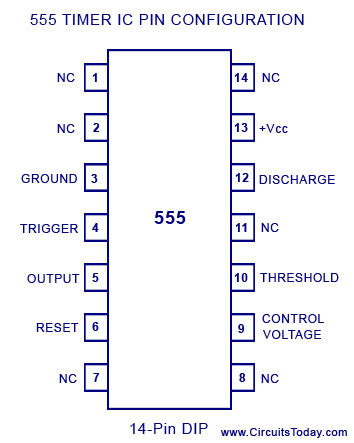Pin 1: Grounded Terminal: All the voltages are measured with respect to the Ground terminal.

Pin 2: Trigger Terminal: The trigger pin is used to feed the trigger input hen the 555 IC is set up as a monostable multivibrator. This pin is an inverting input of a comparator and is responsible for the transition of flip-flop from set to reset. The output of the timer depends on the amplitude of the external trigger pulse applied to this pin. A negative pulse with a dc level greater than Vcc/3 is applied to this terminal. In the negative edge, as the trigger passes through Vcc/3, the output of the lower comparator becomes high and the complimentary of Q becomes zero. Thus the 555 IC output gets a high voltage, and thus a quasi stable state.

Pin 3: Output Terminal: Output of the timer is avail­able at this pin. There are two ways in which a load can be connected to the output terminal. One way is to connect between output pin (pin 3) and ground pin (pin 1) or between pin 3 and supply pin (pin 8). The load connected between output and ground supply pin is called the normally on load and that connected between output and ground pin is called the normally off load.

Pin 4: Reset Terminal: Whenever the timer IC is to be reset or disabled, a negative pulse is applied to pin 4, and thus is named as reset terminal. The output is reset irrespective of the input condition. When this pin is not to be used for reset purpose, it should be connected to + VCC to avoid any possibility of false triggering.

Pin 5: Control Voltage Terminal: The threshold and trigger levels are controlled using this pin. The pulse width of the output waveform is determined by connecting a POT or bringing in an external voltage to this pin. The external voltage applied to this pin can also be used to modulate the output waveform. Thus, the amount of voltage applied in this terminal will decide when the comparator is to be switched, and thus changes the pulse width of the output. When this pin is not used, it should be bypassed to ground through a 0.01 micro Farad to avoid any noise problem.

Pin 6: Threshold Terminal: This is the non-inverting input terminal of comparator 1, which compares the voltage applied to the terminal with a reference voltage of 2/3 VCC. The amplitude of voltage applied to this terminal is responsible for the set state of flip-flop. When the voltage applied in this terminal is greater than 2/3Vcc, the upper comparator switches to +Vsat and the output gets reset.

Pin 7 : Discharge Terminal: This pin is connected internally to the collector of transistor and mostly a capacitor is connected between this terminal and ground. It is called discharge terminal because when transistor saturates, capacitor discharges through the transistor. When the transistor is cut-off, the capacitor charges at a rate determined by the external resistor and capacitor.

Pin 8: Supply Terminal: A supply voltage of + 5 V to + 18 V is applied to this terminal with respect to ground (pin 1).

## 3. 555 Timer Basics

The 555 timer combines a relaxation oscillator, two comparators, an R-S flip-flop, and a discharge capacitor.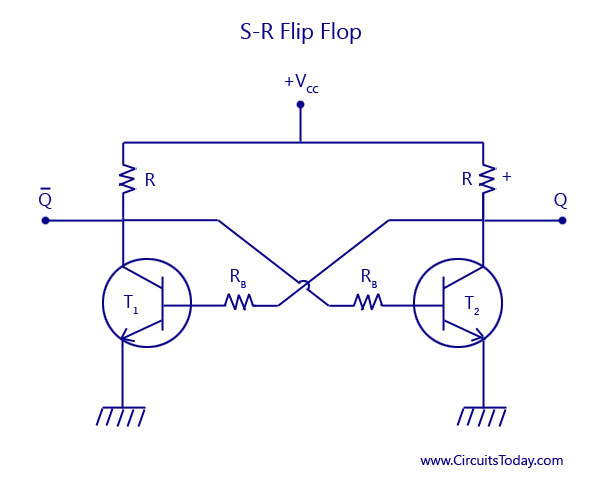S-R-Flip Flop

As shown in the figure, two transistors T1 and T2 are cross coupled. The collector of transistor T1 drives the base of transistor T2 through the resistor Rb2. The collector of transistor T2 drives the base of transistor T1 through resistor Rb1. When one of the transistor is in the saturated state, the other transistor will be in the cut-off state. If we consider the transistor T1 to be saturated, then the collector voltage will be almost zero. Thus there will be a zero base drive for transistor T2 and will go into cut-off state and its collector voltage approaches +Vcc. This voltage is applied to the base of T1 and thus will keep it in saturation.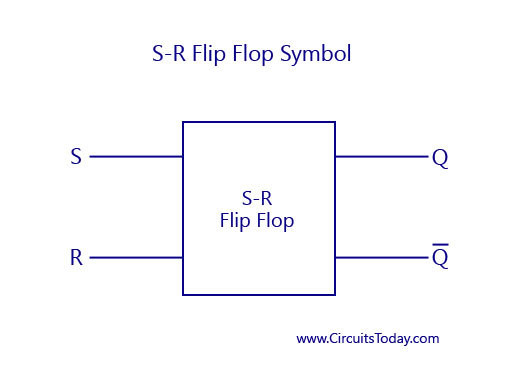S-R Flip Flop Symbol

Now, if we consider the transistor T1 to be in the cut-off state, then the collector voltage of T1 will be equal to +Vcc. This voltage will drive the base of the transistor T2 to saturation. Thus, the saturated collector output of transistor T2 will be almost zero. This value when fedback to the base of the transistor T1 will drive it to cut-off. Thus, the saturation and cut-off value of anyone of the transistors decides the high and low value of Q and its compliment. By adding more components to the circuit, an R-S flip-flop is obtained. R-S flip-flop is a circuit that can set the Q output to highor reset it low. Incidentally, a complementary (opposite) output Q is available from the collector of the other transistor. The schematic symbol for a S-R flip flop is also shown above. The circuit latches in either the Q state or its complimentary state. A high value of S input sets the value of Q to go high. A high value of R input resets the value of Q to low. Output Q remains in a given state until it is triggered into the opposite state.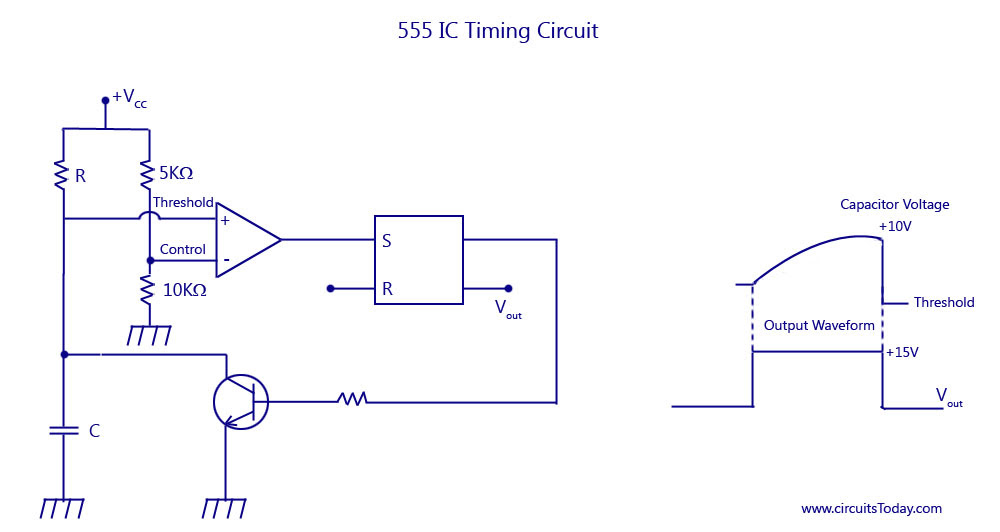555 IC Timing Circuit

### Basic Timing Concept

From the figure above, assuming the output of the S-R flip flop, Q to be high. This high value is passed on to the base of the transistor, and the transistor gets saturated, thus producing a zero voltage at the collector. The capacitor voltage is clamped at ground, that is, the capacitor C is shorted and cannot charge.

The inverting input of the comparator is fed with a control voltage, and the non-inverting input is fed with a threshold voltage. With R-S flip flop set, the saturated transistor holds the threshold voltage at zero. The control voltage, however, is fixed at 2/3 VCC, that is, at 10 volts, because of the voltage divider.

Suppose that a high voltage is applied to the R input. This resets the flip-flop R-Output Q goes lowand the transistor is cut-off. Capacitor C is now free to charge. As this capacitor C charges, the threshold voltage rises. Eventually, the threshold voltage becomes slightly greater than (+ 10 V). The output of the comparator then goes high, forcing the R S flip-flop to set. The high Q output saturates the transistor, and this quickly discharges the capacitor. An exponential rise is across the capacitor C, and a positive going pulse appears at the output Q. Thus capacitor voltage VC is exponential while the output is rectangular. This is shown in the figure above.

## 4. Block Diagram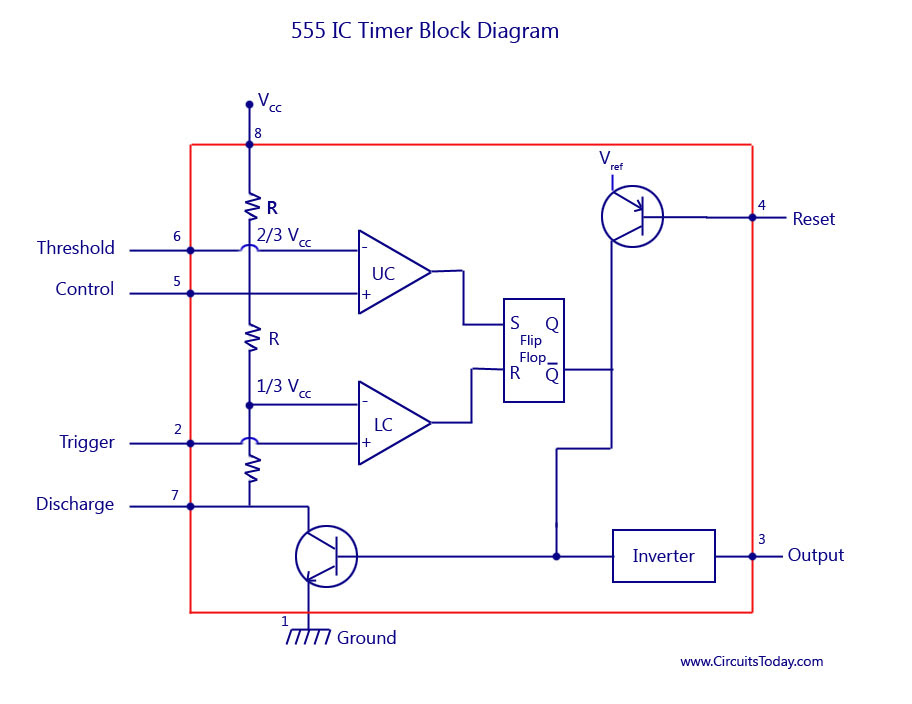555 IC Timer Block Diagram

The block diagram of a 555 timer is shown in the above figure. A 555 timer has two comparators, which are basically 2 op-amps), an R-S flip-flop, two transistors and a resistive network.

• Resistive network consists of three equal resistors and acts as a voltage divider.
• Comparator 1 compares threshold voltage with a reference voltage + 2/3VCC volts.
• Comparator 2 compares the trigger voltage with a reference voltage + 1/3 VCC volts.

Output of both the comparators is supplied to the flip-flop. Flip-flop assumes its state according to the output of the two compa­rators. One of the two transistors is a discharge transistor of which collector is connected to pin 7. This tran­sistor saturates or cuts-off according to the output state of the flip-flop. The saturated transis­tor provides a discharge path to a capacitor con­nected externally. Base of another transistor is connected to a reset terminal. A pulse applied to this terminal resets the whole timer irrespective of any input.

## 5. Working Principle

#### Refer Block Diagram of 555 timer IC given above:

The internal resistors act as a voltage divider network, providing (2/3)Vcc at the non-inverting terminal of the upper comparator and (1/3)Vcc at the inverting terminal of the lower comparator. In most applications, the control input is not used, so that the control voltage equals +(2/3) VCC. Upper comparator has a threshold input (pin 6) and a control input (pin 5). Output of the upper comparator is applied to set (S) input of the flip-flop. Whenever the threshold voltage exceeds the control voltage, the upper comparator will set the flip-flop and its output is high. A high output from the flip-flop when given to the base of the discharge transistor saturates it and thus discharges the transistor that is connected externally to the discharge pin 7. The complementary signal out of the flip-flop goes to pin 3, the output. The output available at pin 3 is low. These conditions will prevail until lower comparator triggers the flip-flop. Even if the voltage at the threshold input falls below (2/3)VCC, that is upper comparator cannot cause the flip-flop to change again. It means that the upper comparator can only force the flip-flop’s output high.

To change the output of flip-flop to low, the voltage at the trigger input must fall below + (1/3) Vcc. When this occurs, lower comparator triggers the flip-flop, forcing its output low. The lowoutput from the flip-flop turns the discharge transistor offand forces the power amplifier to output a high. These conditions will continue independent of the voltage on the trigger input. Lower comparator can only cause the flip-flop to output low.

From the above discussion it is concluded that for the having low output from the timer 555, the voltage on the threshold input must exceed the control voltage or + (2/3)VCC. This also turns the discharge transistor on. To force the output from the timer high, the voltage on the trigger input must drop below +(1/3)VCC. This turns the discharge transistor off.

A voltage may be applied to the control input to change the levels at which the switching occurs. When not in use, a 0.01 nano Farad capacitor should be connected between pin 5 and ground to prevent noise coupled onto this pin from causing false triggering.

Connecting the reset (pin 4) to a logic low will place a high on the output of flip-flop. The discharge transistor will go on and the power amplifier will output a low. This condition will continue until reset is taken high. This allows synchronization or resetting of the circuit’s operation. When not in use, reset should be tied to +VCC.

To know more about NE/SE 555 timer IC check out/download the datasheet. – NE-SE 555 Timer Datasheet

To know more about applications of 555 Timer IC take a look at the following posts:

• 555 TIMER AS AN ASTABLE MULTIVIRATOR
• 555 TIMER AS A MONOSTABLE MULTIVIBRATOR
• 555 TIMER OSCILLATOR
• 555 TIMER – RAMP GENERATOR555 Timer IC Packages

[Lights][grids]